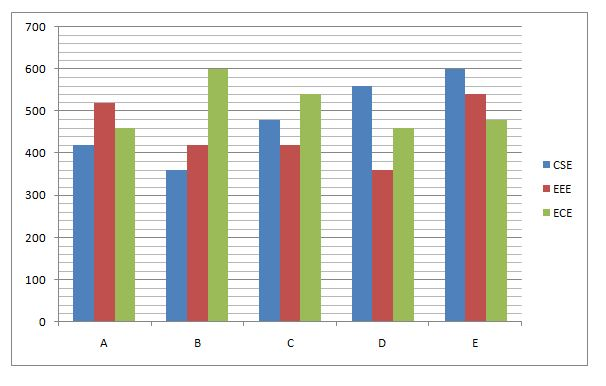# SBI Clerk Pre Quantitative Aptitude (Day-46)

Dear Aspirants, Our IBPS Guide team is providing new series of Quantitative Aptitude Questions for SBI Clerk Prelims 2020 so the aspirants can practice it on a daily basis. These questions are framed by our skilled experts after understanding your needs thoroughly. Aspirants can practice these new series questions daily to familiarize with the exact exam pattern and make your preparation effective.

Start Quiz

Ensure Your Ability Before the Exam – Take SBI Clerk 2020 Prelims Free Mock Test

Wrong Number Series

Directions (1 – 5): Find out the wrong number in the following number series?

1) 12, 39, 164, 500, 1236, 2567

a) 164

b) 500

c) 1236

d) 2567

e) 39

2) 251, 276, 257, 271, 261, 266

a) 251

b) 276

c) 257

d) 271

e) 261

3) 28, 35, 42, 70, 98, 133

a) 35

b) 42

c) 70

d) 98

e) 28

4) 9, 26, 65, 120, 217, 342

a) 9

b) 26

c) 65

d) 120

e) None of these

5) 3, 12, 33, 72, 135, 230

a) 230

b) 135

c) 72

d) 33

e) None of these

Data Interpretation

Direction (6 – 10): Study the following information carefully and answer the given questions?

The given bar graph shows the number of students in three different departments from four different colleges.6) The average number of students from E in all the departments together is approximately what percent more than that of the average number of students from D in all the departments together?

a) 13%

b) 15%

c) 17%

d) 19%

e) 11%

7) What is the difference between the number of students from A, B and D together in EEE departments and the number of students from B, C and E in ECE departments together?

a) 280

b) 320

c) 260

d) 300

e) 260

8) The number of students in CSE department from C is approximately what percent of the total number of students in CSE departments from all the colleges together?

a) 20%

b) 23%

c) 27%

d) 17%

e) 13%

9) What is average number of students in ECE from all the colleges together?

a) 510

b) 508

c) 512

d) 514

e) 506

10) What is the ratio of the number of students from A, B and D together in ECE department to number of students from B, C and E together in EEE departments?

a) 71:63

b) 72:65

c) 76:69

d) 78:71

e) None of these

Directions (1-5):

12 + 33 = 39

39 + 53 = 164

164 + 73 = 507 (not 500)

507 + 93 = 1236

1236 + 113 = 2567

251 + 25 = 276

276 – 20 = 256 (not 257)

256 + 15 = 271

271 – 10 = 261

261 + 5 = 266

28 + 7 = 35

35 + 14 = 49 (not 42)

49 + 21 = 70

70 + 28 = 98

98 + 35 = 133

23 + 1 = 9

33 – 1 = 26

43 + 1 = 65

53 – 1 = 124 (not 120)

63 + 1 = 217

73 – 1 = 342

13 + 2 = 3

23 + 4 = 12

33 + 6 = 33

43 + 8 = 72

53 + 10 = 135

63 + 12 = 228 (not 230)

Directions (6-10):

Average students from E=600 + 540 + 480/3=540

Average students from D=560 + 360 + 460/3=460

Required percentage = [(540 – 460)/460] * 100=17%

Number of students from A, B and D in EEE = (520 + 420 + 360) = 1300

Number of students from B, C and E in ECE = (600 + 540 + 480) = 1620

Difference = 1620 – 1300 = 320

Required percentage

= [480/(420 + 360 + 480 + 560 + 600)] * 100

= 20%

Average = (460 + 600 + 540 + 460 + 480)/5

=508

Required ratio = (460 + 600 + 460) : (420 + 420 + 540)

=1520 : 1380

=76 : 69

 Check Here to View SBI Clerk Prelims 2020 Quantitative Aptitude Questions Day 45 Day 44 Day 43 Click Here for SBI Clerk 2020 – Detailed Exam Notification